5.2 Analysis By looking to the graphs in Figures 6 and 7 which respectively represent powers in primary and secondary coild, it was hard to detect a single equation which would describe how does it behave. It was neither a single quadratic nor a single linear equation. In my opinion, the power in both graphs was decreasing in a very small rate from 5 to 100 Hz and that decrease could be described by a linear equations. As it can be seen in the graphs, equations of power from 5 to 100 Hz in form of y=mx + b are represented. The second thing that I have observed is that powers from 100 to 1000 Hz are decreasing in a much bigger rate, but still linearly. In my opinion, there are several ways how to describe such behaviour of the transformers: Impedance. The impedance is influenced by both resistance and the reactance. I assume that the resistance of the system does not change while we are changing the frequency. On the other hand the reactance is directly proportional to the frequency in AC circuit. The reactance formula is given by: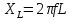, where XL – reactance, f – frequency, L - inductance. Therefore: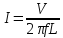, where I – current, V – voltage. Even though, we can see from the formula that higher frequency influences a smaller current, it does not explain why does the current decrease linearly, but not by inverse function as the above formula suggest. Firstly, it happens because, as we can see from the Figure 4, voltage does not stay the same throughout the process. Secondly, another phenomenon, in my opinion, has an influence in this experiment: Skin effect. Skin effect is basically the tendency of alternating current to become distributed densest near the surface of conductor as the frequency of a current increases. As the current flows denser near the surface of a conductor, the resistance of the material increases, because the cross-section area decreases: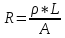, where ρ-resistivity of a material, L – length of a material, A – cross-section area. Even though these two phenomena (impedance and skin effect) with the decrease of voltage explain why does power decrease, it does not explain why does the rate of decrease differs in intervals from 5 to 100 Hz and from 100 to 1000 Hz. I can make an assumption that the designers of this transformers have solved how to minimize the losses due to frequency change from 5 to 100 Hz which are the nearest to 50 Hz (which is the usual current being used in Europe). It is a very logical design decision, as people do not usually use frequencies of current higher than 100 Hz. Figure 8 shows a graph of efficiency from the frequency. As we can see, it is a cubic function of form bx3 + cx2 +dx + a. From this graph we can see that the efficiency from 5 to 100 Hz almost does not change. As it could already be predicted from the power functions, the efficiency almost does not change in the interval from 5 to 100 Hz and is equal to about 80 %. From 100 Hz the slope of a function becomes negative and starts to decrease even more as x approaches infinity. 5.2.1 Uncertainties The uncertainties of power, efficiency and frequency can be seen in a figure 9 below. Figure 9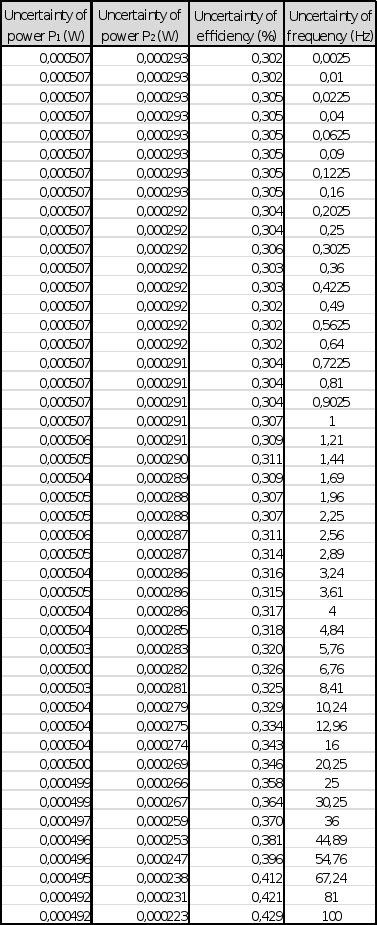To calculate the uncertainties of power and efficiency I have used formulas: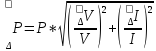Where ΔP – uncertainty of Power, P – Power, ΔV = systematic error of voltage, V – voltage, ΔI – systematic error of current, I – current.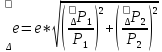Where Δe – uncertainty of efficiency, e – efficiency, ΔP1 = uncertainty of input power, P1 – input power, ΔP2 – uncertainty of output power, P2 – output power. One of the most important things to take into account was the rate at which measurements were taken. As it is mentioned before, both Nova computers could take 10 000 measurements per second which is enough for measuring the current of low frequencies. On the other hand, 10 000 measurements per second might not be sufficient for higher frequencies, such as 1 000 Hz, because then the percentage uncertainty is as high as 10 %. Therefore, by considering the data I have gathered, I have decided to take frequency uncertainties into account by using this formula: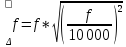which is equivalent to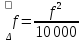As we can see in the figure 6 and 7 above which represent power functions from frequency the uncertainties in y axis (power uncertainties) are relatively small and many of them do not fit in the straight line graphs. Only by considering these uncertainties the measurements could be said to very inaccurate but the frequency uncertainties are very important while considering this. As we can see, the uncertainties in x axis are relatively big and the straight line graph fits in the most of the error bars of it. Latter uncertainties make the interpretation of a data more justifiable. By analysing figure 8 which represents efficiency from frequency function we can see that the uncertainties in y axis have more meaning than the ones in power functions as the formers are relatively bigger. With addition to the uncertainties in x axis we can see that the polynomial efficiency function fits in the most of the error bars. 6. Conclusion and evaluation 6.1 Conclusion During the investigation several things were found out: The power in primary and secondary coils is highest in range from 5 to 100 Hz which are the nearest points to the frequency of 50 Hz for which this transformer was designed to work. The power in both primary and secondary coils drops linearly in range from 100 to 1 000 Hz. Assumption can be made that such dependency continues after 1 000 Hz also. The efficiency of transformer does not highly depend on the frequency in range from 5 to 100 Hz as it stays almost constant – about 80 %. The efficiency drops in increasing rates in range from 100 to 1 000 Hz. Assumption can be made that such dependency continues after 1 000 Hz also. Conclusion can be made that transformers similar to the one which was tested in experiments can be easily used in frequencies that are close to the ones that they were built for. For example, European transformer built for the currents of 50 Hz can be easily used in North America where the frequency of the current is 60 Hz. It is not advisable to use such transformers in frequencies bigger than 100 Hz as both the power and the efficiency of a transformer starts to drop. 6.2 Evaluation Even though the investigation can be said to be successful, there are several points on how could this research could be improved: The data might have been much more accurate if I had used bigger power loads for the transformer. It was originally made to work in voltages of 230 V while the voltage I have used in the primary coil was 10 V. On the other hand, the data was quite logical and the voltage ratio is19 times – the same as it is written on the label of the transformer. The measuring the devices could have been much more accurate. It is difficult to say whether the voltmeters and ampere-meters gathered accurate data but it is obvious that the uncertainty of frequency is too big. As the frequencies increased to as high as 1 000 Hz the uncertainty of frequency increased to 10 per cent which does not give very accurate data. That is because as we have a sinusoid of either voltage of current, the devices might not detect the peak which are the most important values for the research but rather choose the values that are smaller than the values of the peaks. During the research more transformers could be used. One device might not show universal results, because other transformers might perform a little bit differently in the conditions that were created during the investigation. Some external factors, such as the resistance of wires or the temperature of devices might have slightly influenced the results of this investigation. If the all data could be taken into account, the measurements could be much more accurate. 7. Bibliography Inductive Reactance Formulae & Calculations. http://www.radio-electronics.com/info/formulae/inductance/inductor-inductive-reactance-formulae-calculations.php Last accessed 30 September 2014. Formulas for calculating uncertainties. http://webpages.ursinus.edu/lriley/ref/unc/unc.html Last accessed 20 December 2014. Download 0.53 Mb.Share with your friends: